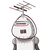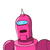# If x is 10% less than yo then ythan xby what per percentageyis more​

If x is 10% less than yo then y
than x
by what per percentage
y
is more​

### 2 thoughts on “If x is 10% less than yo then y<br />than x<br />by what per percentage<br />y<br />is more​”

1.aggsuahxjanxjajsknxsnakosnx sanakzos

2.100/9%

Step-by-step explanation:

Let y be 100.

Then x will be 90.

We have to compare wrt x.

So,

%more=(100-90)x100/90=100/9%